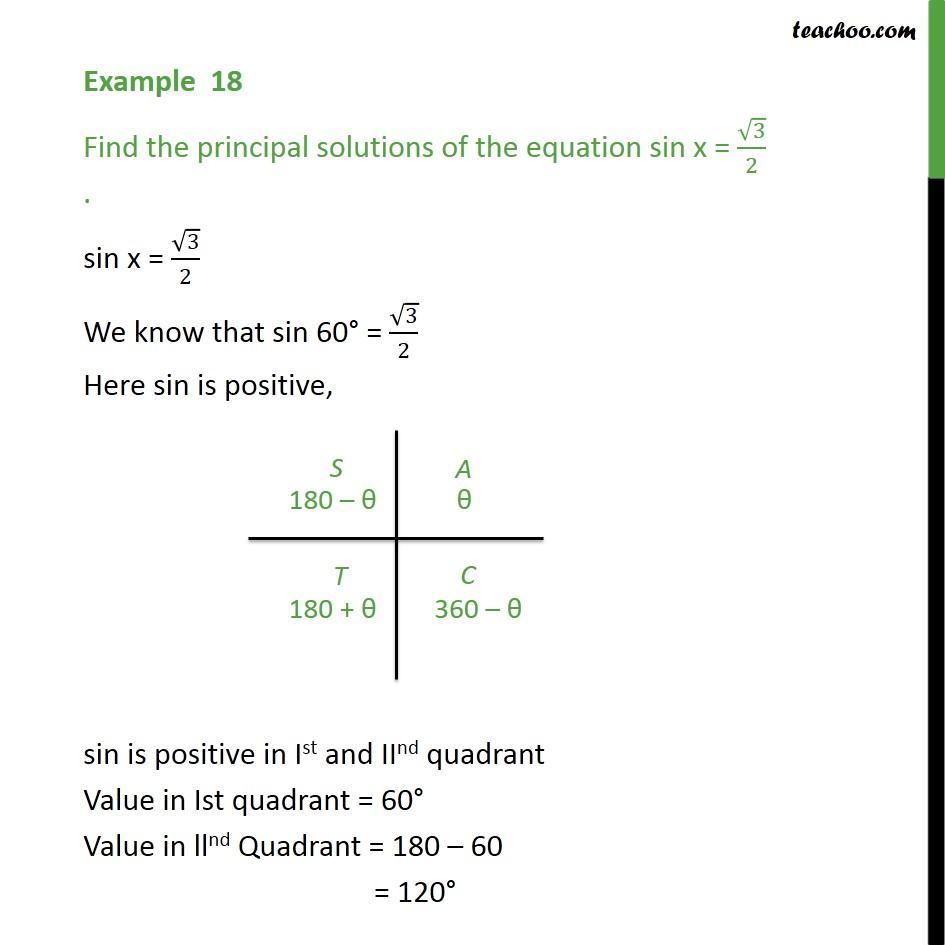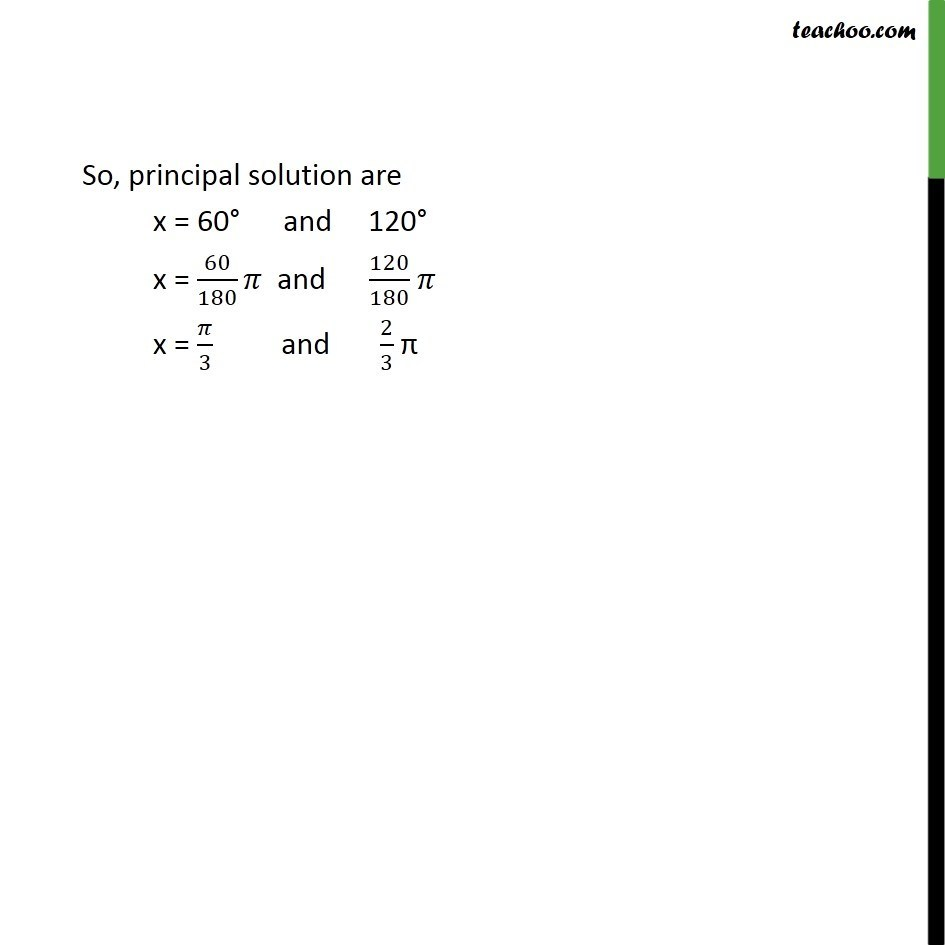Subscribe to our Youtube Channel - https://you.tube/teachoo

1. Chapter 3 Class 11 Trigonometric Functions
2. Concept wise
3. Finding Principal solutions

Transcript

Example 18 Find the principal solutions of the equation sin x = √3/2 . sin x = √3/2 We know that sin 60° = √3/2 Here sin is positive, sin is positive in Ist and IInd quadrant Value in Ist quadrant = 60° Value in llnd Quadrant = 180 – 60 = 120° So, principal solution are x = 60° and 120° x = 60/180 𝜋 and 120/180 𝜋 x = 𝜋/3 and 2/3 π

Finding Principal solutions